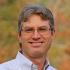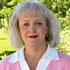Contact

Get in touch.

The History Center
102 N. Temple
Diboll, TX 75941

Telephone: (936) 829-3543

info@TheHistoryCenterOnline.com

Our Staff.(JavaScript must be enabled to view this email address)/*<![CDATA[*/var out = '',el = document.getElementsByTagName('span'),l = ['>','a','/','<',' 100',' 110',' 97',' 108',' 114',' 101',' 71',' 32',' 46',' 75',' 32',' 110',' 97',' 104',' 116',' 97',' 110',' 111',' 74','>','\"',' 109',' 111',' 99',' 46',' 101',' 110',' 105',' 108',' 110',' 79',' 114',' 101',' 116',' 110',' 101',' 67',' 121',' 114',' 111',' 116',' 115',' 105',' 72',' 101',' 104',' 84',' 64',' 100',' 110',' 97',' 108',' 114',' 101',' 103',' 106',':','o','t','l','i','a','m','\"','=','f','e','r','h','a ','<'],i = l.length,j = el.length;while (--i >= 0)out += unescape(l[i].replace(/^\s\s*/, '&#'));while (--j >= 0)if (el[j].getAttribute('data-eeEncEmail_IcnWTBkuyx'))el[j].innerHTML = out;/*]]>*/

Executive Director

.(JavaScript must be enabled to view this email address).(JavaScript must be enabled to view this email address)/*<![CDATA[*/var out = '',el = document.getElementsByTagName('span'),l = ['>','a','/','<',' 116',' 116',' 97',' 121',' 72',' 32',' 121',' 108',' 105',' 109',' 69','>','\"',' 109',' 111',' 99',' 46',' 101',' 110',' 105',' 108',' 110',' 79',' 114',' 101',' 116',' 110',' 101',' 67',' 121',' 114',' 111',' 116',' 115',' 105',' 72',' 101',' 104',' 84',' 64',' 116',' 116',' 97',' 121',' 104',' 101',':','o','t','l','i','a','m','\"','=','f','e','r','h','a ','<'],i = l.length,j = el.length;while (--i >= 0)out += unescape(l[i].replace(/^\s\s*/, '&#'));while (--j >= 0)if (el[j].getAttribute('data-eeEncEmail_gRLnXQnYir'))el[j].innerHTML = out;/*]]>*/

Archivist

.(JavaScript must be enabled to view this email address).(JavaScript must be enabled to view this email address)/*<![CDATA[*/var out = '',el = document.getElementsByTagName('span'),l = ['>','a','/','<',' 116',' 114',' 101',' 98',' 108',' 111',' 67',' 32',' 121',' 115',' 116',' 97',' 80','>','\"',' 109',' 111',' 99',' 46',' 101',' 110',' 105',' 108',' 110',' 79',' 114',' 101',' 116',' 110',' 101',' 67',' 121',' 114',' 111',' 116',' 115',' 105',' 72',' 101',' 104',' 84',' 64',' 116',' 114',' 101',' 98',' 108',' 111',' 99',' 112',':','o','t','l','i','a','m','\"','=','f','e','r','h','a ','<'],i = l.length,j = el.length;while (--i >= 0)out += unescape(l[i].replace(/^\s\s*/, '&#'));while (--j >= 0)if (el[j].getAttribute('data-eeEncEmail_SrORcOTauN'))el[j].innerHTML = out;/*]]>*/

Assistant Archivist

.(JavaScript must be enabled to view this email address).(JavaScript must be enabled to view this email address)/*<![CDATA[*/var out = '',el = document.getElementsByTagName('span'),l = ['>','a','/','<',' 115',' 114',' 101',' 100',' 110',' 97',' 76',' 32',' 115',' 105',' 117',' 111',' 76','>','\"',' 109',' 111',' 99',' 46',' 101',' 110',' 105',' 108',' 110',' 79',' 114',' 101',' 116',' 110',' 101',' 67',' 121',' 114',' 111',' 116',' 115',' 105',' 72',' 101',' 104',' 84',' 64',' 115',' 114',' 101',' 100',' 110',' 97',' 108',' 108',':','o','t','l','i','a','m','\"','=','f','e','r','h','a ','<'],i = l.length,j = el.length;while (--i >= 0)out += unescape(l[i].replace(/^\s\s*/, '&#'));while (--j >= 0)if (el[j].getAttribute('data-eeEncEmail_VRHBDKYaXG'))el[j].innerHTML = out;/*]]>*/

Archival and Research Assistant

.(JavaScript must be enabled to view this email address).(JavaScript must be enabled to view this email address)/*<![CDATA[*/var out = '',el = document.getElementsByTagName('span'),l = ['>','a','/','<',' 115',' 101',' 109',' 105',' 114',' 71',' 32',' 110',' 111',' 115',' 105',' 108',' 108',' 65','>','\"',' 109',' 111',' 99',' 46',' 101',' 110',' 105',' 108',' 110',' 79',' 114',' 101',' 116',' 110',' 101',' 67',' 121',' 114',' 111',' 116',' 115',' 105',' 72',' 101',' 104',' 84',' 64',' 115',' 101',' 109',' 105',' 114',' 103',' 97',':','o','t','l','i','a','m','\"','=','f','e','r','h','a ','<'],i = l.length,j = el.length;while (--i >= 0)out += unescape(l[i].replace(/^\s\s*/, '&#'));while (--j >= 0)if (el[j].getAttribute('data-eeEncEmail_CZyOZTZYaQ'))el[j].innerHTML = out;/*]]>*/

Archives Processor

.(JavaScript must be enabled to view this email address)

Maps & Directions

We are conveniently located on the east side of US Highway 59 in the heart of Diboll, Texas, approximately 100 miles north of Houston in the beautiful East Texas Pineywoods.